INSTRUCTORSCarleen EatonGrant FraserDr. Carleen Eaton

Graphing Inequalities

Slide Duration:

Section 1: Equations and Inequalities
Expressions and Formulas

22m 23s

Intro
0:00
Order of Operations
0:19
Variable
0:27
Algebraic Expression
0:46
Term
0:57
Example: Algebraic Expression
1:25
Evaluate Inside Grouping Symbols
1:55
Evaluate Powers
2:30
Multiply/Divide Left to Right
2:55
3:35
Monomials
4:40
Examples of Monomials
4:52
Constant
5:27
Coefficient
5:46
Degree
6:25
Power
7:15
Polynomials
8:02
Examples of Polynomials
8:24
Binomials, Trinomials, Monomials
8:53
Term
9:21
Like Terms
10:02
Formulas
11:00
Example: Pythagorean Theorem
11:15
Example 1: Evaluate the Algebraic Expression
11:50
Example 2: Evaluate the Algebraic Expression
14:38
Example 3: Area of a Triangle
19:11
Example 4: Fahrenheit to Celsius
20:41
Properties of Real Numbers

20m 15s

Intro
0:00
Real Numbers
0:07
Number Line
0:15
Rational Numbers
0:46
Irrational Numbers
2:24
Venn Diagram of Real Numbers
4:03
Irrational Numbers
5:00
Rational Numbers
5:19
Real Number System
5:27
Natural Numbers
5:32
Whole Numbers
5:53
Integers
6:19
Fractions
6:46
Properties of Real Numbers
7:15
Commutative Property
7:34
Associative Property
8:07
Identity Property
9:04
Inverse Property
9:53
Distributive Property
11:03
Example 1: What Set of Numbers?
12:21
Example 2: What Properties Are Used?
13:56
Example 3: Multiplicative Inverse
16:00
Example 4: Simplify Using Properties
17:18
Solving Equations

19m 10s

Intro
0:00
Translations
0:06
Verbal Expressions and Algebraic Expressions
0:13
Example: Sum of Two Numbers
0:19
Example: Square of a Number
1:33
Properties of Equality
3:20
Reflexive Property
3:30
Symmetric Property
3:42
Transitive Property
4:01
5:01
Subtraction Property
5:37
Multiplication Property
6:02
Division Property
6:30
Solving Equations
6:58
Example: Using Properties
7:18
Solving for a Variable
8:25
Example: Solve for Z
8:34
Example 1: Write Algebraic Expression
10:15
Example 2: Write Verbal Expression
11:31
Example 3: Solve the Equation
14:05
Example 4: Simplify Using Properties
17:26
Solving Absolute Value Equations

17m 31s

Intro
0:00
Absolute Value Expressions
0:09
Distance from Zero
0:18
Example: Absolute Value Expression
0:24
Absolute Value Equations
1:50
Example: Absolute Value Equation
2:00
Example: Isolate Expression
3:13
No Solution
3:46
Empty Set
3:58
Example: No Solution
4:12
Number of Solutions
4:46
Check Each Solution
4:57
Example: Two Solutions
5:05
Example: No Solution
6:18
Example: One Solution
6:28
Example 1: Evaluate for X
7:16
Example 2: Write Verbal Expression
9:08
Example 3: Solve the Equation
12:18
Example 4: Simplify Using Properties
13:36
Solving Inequalities

17m 14s

Intro
0:00
Properties of Inequalities
0:08
0:17
Example: Using Numbers
0:30
Subtraction Property
1:03
Example: Using Numbers
1:19
Multiplication Properties
1:44
C>0 (Positive Number)
1:50
Example: Using Numbers
2:05
C<0 (Negative Number)
2:40
Example: Using Numbers
3:10
Division Properties
4:11
C>0 (Positive Number)
4:15
Example: Using Numbers
4:27
C<0 (Negative Number)
5:21
Example: Using Numbers
5:32
Describing the Solution Set
6:10
Example: Set Builder Notation
6:26
Example: Graph (Closed Circle)
7:08
Example: Graph (Open Circle)
7:30
Example 1: Solve the Inequality
7:58
Example 2: Solve the Inequality
9:06
Example 3: Solve the Inequality
10:10
Example 4: Solve the Inequality
13:12
Solving Compound and Absolute Value Inequalities

25m

Intro
0:00
Compound Inequalities
0:08
And and Or
0:13
Example: And
0:22
Example: Or
1:12
And Inequality
1:41
Intersection
1:49
Example: Numbers
2:08
Example: Inequality
2:43
Or Inequality
4:35
Example: Union
4:45
Example: Inequality
5:53
Absolute Value Inequalities
7:19
Definition of Absolute Value
7:33
Examples: Compound Inequalities
8:30
Example: Complex Inequality
12:21
Example 1: Solve the Inequality
12:54
Example 2: Solve the Inequality
17:21
Example 3: Solve the Inequality
18:54
Example 4: Solve the Inequality
22:15
Section 2: Linear Relations and Functions
Relations and Functions

32m 5s

Intro
0:00
Coordinate Plane
0:20
X-Coordinate and Y-Coordinate
0:30
Example: Coordinate Pairs
0:37
1:20
Relations
2:14
Domain and Range
2:19
Set of Ordered Pairs
2:29
As a Table
2:51
Functions
4:21
One Element in Range
4:32
Example: Mapping
4:43
Example: Table and Map
6:26
One-to-One Functions
8:01
Example: One-to-One
8:22
Example: Not One-to-One
9:18
Graphs of Relations
11:01
Discrete and Continuous
11:12
Example: Discrete
11:22
Example: Continous
12:30
Vertical Line Test
14:09
Example: S Curve
14:29
Example: Function
16:15
Equations, Relations, and Functions
17:03
Independent Variable and Dependent Variable
17:16
Function Notation
19:11
Example: Function Notation
19:23
Example 1: Domain and Range
20:51
Example 2: Discrete or Continous
23:03
Example 3: Discrete or Continous
25:53
Example 4: Function Notation
30:05
Linear Equations

14m 46s

Intro
0:00
Linear Equations and Functions
0:07
Linear Equation
0:19
Example: Linear Equation
0:29
Example: Linear Function
1:07
Standard Form
2:02
Integer Constants with No Common Factor
2:08
Example: Standard Form
2:27
Graphing with Intercepts
4:05
X-Intercept and Y-Intercept
4:12
Example: Intercepts
4:26
Example: Graphing
5:14
Example 1: Linear Function
7:53
Example 2: Linear Function
9:10
Example 3: Standard Form
10:04
Example 4: Graph with Intercepts
12:25
Slope

23m 7s

Intro
0:00
Definition of Slope
0:07
Change in Y / Change in X
0:26
Example: Slope of Graph
0:37
Interpretation of Slope
3:07
Horizontal Line (0 Slope)
3:13
Vertical Line (Undefined Slope)
4:52
Rises to Right (Positive Slope)
6:36
Falls to Right (Negative Slope)
6:53
Parallel Lines
7:18
Example: Not Vertical
7:30
Example: Vertical
7:58
Perpendicular Lines
8:31
Example: Perpendicular
8:42
Example 1: Slope of Line
10:32
Example 2: Graph Line
11:45
Example 3: Parallel to Graph
13:37
Example 4: Perpendicular to Graph
17:57
Writing Linear Functions

23m 5s

Intro
0:00
Slope Intercept Form
0:11
m and b
0:28
Example: Graph Using Slope Intercept
0:43
Point Slope Form
2:41
Relation to Slope Formula
3:03
Example: Point Slope Form
4:36
Parallel and Perpendicular Lines
6:28
Review of Parallel and Perpendicular Lines
6:31
Example: Parallel
7:50
Example: Perpendicular
9:58
Example 1: Slope Intercept Form
11:07
Example 2: Slope Intercept Form
13:07
Example 3: Parallel
15:49
Example 4: Perpendicular
18:42
Special Functions

31m 5s

Intro
0:00
Step Functions
0:07
Example: Apple Prices
0:30
Absolute Value Function
4:55
Example: Absolute Value
5:05
Piecewise Functions
9:08
Example: Piecewise
9:27
Example 1: Absolute Value Function
14:00
Example 2: Absolute Value Function
20:39
Example 3: Piecewise Function
22:26
Example 4: Step Function
25:25
Graphing Inequalities

21m 42s

Intro
0:00
Graphing Linear Inequalities
0:07
0:19
Using Test Points
0:32
Graph Corresponding Linear Function
0:46
Dashed or Solid Lines
0:59
Use Test Point
1:21
Example: Linear Inequality
1:58
Graphing Absolute Value Inequalities
4:50
Graph Corresponding Equations
4:59
Use Test Point
5:20
Example: Absolute Value Inequality
5:38
Example 1: Linear Inequality
9:17
Example 2: Linear Inequality
11:56
Example 3: Linear Inequality
14:29
Example 4: Absolute Value Inequality
17:06
Section 3: Systems of Equations and Inequalities
Solving Systems of Equations by Graphing

17m 13s

Intro
0:00
Systems of Equations
0:09
Example: Two Equations
0:24
Solving by Graphing
0:53
Point of Intersection
1:09
Types of Systems
2:29
Independent (Single Solution)
2:34
Dependent (Infinite Solutions)
3:05
Inconsistent (No Solution)
4:23
Example 1: Solve by Graphing
5:20
Example 2: Solve by Graphing
9:10
Example 3: Solve by Graphing
12:27
Example 4: Solve by Graphing
14:54
Solving Systems of Equations Algebraically

23m 53s

Intro
0:00
Solving by Substitution
0:08
Example: System of Equations
0:36
Solving by Multiplication
7:22
Extra Step of Multiplying
7:38
Example: System of Equations
8:00
Inconsistent and Dependent Systems
11:14
Variables Drop Out
11:48
Inconsistent System (Never True)
12:01
Constant Equals Constant
12:53
Dependent System (Always True)
13:11
Example 1: Solve Algebraically
13:58
Example 2: Solve Algebraically
15:52
Example 3: Solve Algebraically
17:54
Example 4: Solve Algebraically
21:40
Solving Systems of Inequalities By Graphing

27m 12s

Intro
0:00
Solving by Graphing
0:08
Graph Each Inequality
0:25
Overlap
0:35
Corresponding Linear Equations
1:03
Test Point
1:23
Example: System of Inequalities
1:51
No Solution
7:06
Empty Set
7:26
Example: No Solution
7:34
Example 1: Solve by Graphing
10:27
Example 2: Solve by Graphing
13:30
Example 3: Solve by Graphing
17:19
Example 4: Solve by Graphing
23:23
Solving Systems of Equations in Three Variables

28m 53s

Intro
0:00
Solving Systems in Three Variables
0:17
Triple of Values
0:31
Example: Three Variables
0:56
Number of Solutions
5:55
One Solution
6:08
No Solution
6:24
Infinite Solutions
7:06
Example 1: Solve 3 Variables
7:59
Example 2: Solve 3 Variables
13:50
Example 3: Solve 3 Variables
19:54
Example 4: Solve 3 Variables
25:50
Section 4: Matrices
Basic Matrix Concepts

11m 34s

Intro
0:00
What is a Matrix
0:26
Brackets
0:46
Designation
1:21
Element
1:47
Matrix Equations
1:59
Dimensions
2:27
Rows (m) and Columns (n)
2:37
Examples: Dimensions
2:43
Special Matrices
4:22
Row Matrix
4:32
Column Matrix
5:00
Zero Matrix
6:00
Equal Matrices
6:30
Example: Corresponding Elements
6:36
Example 1: Matrix Dimension
8:12
Example 2: Matrix Dimension
9:03
Example 3: Zero Matrix
9:38
Example 4: Row and Column Matrix
10:26
Matrix Operations

21m 36s

Intro
0:00
0:18
Same Dimensions
0:25
1:04
Matrix Subtraction
3:42
Same Dimensions
3:48
Example: Subtracting Matrices
4:04
Scalar Multiplication
6:08
Scalar Constant
6:24
Example: Multiplying Matrices
6:32
Properties of Matrix Operations
8:23
Commutative Property
8:41
Associative Property
9:08
Distributive Property
9:44
10:24
Example 2: Matrix Subtraction
11:58
Example 3: Scalar Multiplication
14:23
Example 4: Matrix Properties
16:09
Matrix Multiplication

29m 36s

Intro
0:00
Dimension Requirement
0:17
n = p
0:24
Resulting Product Matrix (m x q)
1:21
Example: Multiplication
1:54
Matrix Multiplication
3:38
Example: Matrix Multiplication
4:07
Properties of Matrix Multiplication
10:46
Associative Property
11:00
Associative Property (Scalar)
11:28
Distributive Property
12:06
Distributive Property (Scalar)
12:30
Example 1: Possible Matrices
13:31
Example 2: Multiplying Matrices
17:08
Example 3: Multiplying Matrices
20:41
Example 4: Matrix Properties
24:41
Determinants

33m 13s

Intro
0:00
What is a Determinant
0:13
Square Matrices
0:23
Vertical Bars
0:41
Determinant of a 2x2 Matrix
1:21
Second Order Determinant
1:37
Formula
1:45
Example: 2x2 Determinant
1:58
Determinant of a 3x3 Matrix
2:50
Expansion by Minors
3:08
Third Order Determinant
3:19
Expanding Row One
4:06
Example: 3x3 Determinant
6:40
Diagonal Method for 3x3 Matrices
13:24
Example: Diagonal Method
13:36
Example 1: Determinant of 2x2
18:59
Example 2: Determinant of 3x3
20:03
Example 3: Determinant of 3x3
25:35
Example 4: Determinant of 3x3
29:22
Cramer's Rule

28m 25s

Intro
0:00
System of Two Equations in Two Variables
0:16
One Variable
0:50
Determinant of Denominator
1:14
Determinants of Numerators
2:23
Example: System of Equations
3:34
System of Three Equations in Three Variables
7:06
Determinant of Denominator
7:17
Determinants of Numerators
7:52
Example 1: Two Equations
8:57
Example 2: Two Equations
13:21
Example 3: Three Equations
17:11
Example 4: Three Equations
23:43
Identity and Inverse Matrices

22m 25s

Intro
0:00
Identity Matrix
0:13
Example: 2x2 Identity Matrix
0:30
Example: 4x4 Identity Matrix
0:50
Properties of Identity Matrices
1:24
Example: Multiplying Identity Matrix
2:52
Matrix Inverses
5:30
Writing Matrix Inverse
6:07
Inverse of a 2x2 Matrix
6:39
Example: 2x2 Matrix
7:31
Example 1: Inverse Matrix
10:18
Example 2: Find the Inverse Matrix
13:04
Example 3: Find the Inverse Matrix
17:53
Example 4: Find the Inverse Matrix
20:44
Solving Systems of Equations Using Matrices

22m 32s

Intro
0:00
Matrix Equations
0:11
Example: System of Equations
0:21
Solving Systems of Equations
4:01
Isolate x
4:16
Example: Using Numbers
5:10
Multiplicative Inverse
5:54
Example 1: Write as Matrix Equation
7:18
Example 2: Use Matrix Equations
9:12
Example 3: Use Matrix Equations
15:06
Example 4: Use Matrix Equations
19:35
Section 5: Quadratic Functions and Inequalities

31m 48s

Intro
0:00
0:12
A is Zero
0:27
Example: Parabola
0:45
Properties of Parabolas
2:08
Axis of Symmetry
2:11
Vertex
2:32
Example: Parabola
2:48
Minimum and Maximum Values
9:02
Positive or Negative
9:28
Upward or Downward
9:58
Example: Minimum
10:31
Example: Maximum
11:16
Example 1: Axis of Symmetry, Vertex, Graph
12:41
Example 2: Axis of Symmetry, Vertex, Graph
17:25
Example 3: Minimum or Maximum
21:47
Example 4: Minimum or Maximum
27:09

27m 3s

Intro
0:00
0:16
Standard Form
0:18
0:47
Solving by Graphing
1:41
Roots (x-Intercepts)
1:48
Example: Number of Solutions
2:12
Estimating Solutions
9:23
Example: Integer Solutions
9:30
Example: Estimating
9:53
Example 1: Solve by Graphing
10:52
Example 2: Solve by Graphing
15:10
Example 1: Solve by Graphing
17:50
Example 1: Solve by Graphing
20:54

19m 53s

Intro
0:00
Factoring Techniques
0:15
Greatest Common Factor (GCF)
0:37
Difference of Two Squares
1:48
Perfect Square Trinomials
2:30
General Trinomials
3:09
Zero Product Rule
5:22
Example: Zero Product
5:53
Example 1: Solve by Factoring
7:46
Example 1: Solve by Factoring
9:48
Example 1: Solve by Factoring
12:34
Example 1: Solve by Factoring
15:28
Imaginary and Complex Numbers

35m 45s

Intro
0:00
Properties of Square Roots
0:10
Product Property
0:26
Example: Product Property
0:56
Quotient Property
2:17
Example: Quotient Property
2:35
Imaginary Numbers
3:12
Imaginary i
3:51
Examples: Imaginary Number
4:22
Complex Numbers
7:23
Real Part and Imaginary Part
7:33
Examples: Complex Numbers
7:57
Equality
9:37
Example: Equal Complex Numbers
9:52
10:12
10:25
Complex Plane
13:32
Horizontal Axis (Real)
13:49
Vertical Axis (Imaginary)
13:59
Example: Labeling
14:11
Multiplication
15:57
Example: FOIL Method
16:03
Division
18:37
Complex Conjugates
18:45
Conjugate Pairs
19:10
Example: Dividing Complex Numbers
20:00
Example 1: Simplify Complex Number
24:50
Example 2: Simplify Complex Number
27:56
Example 3: Multiply Complex Numbers
29:27
Example 3: Dividing Complex Numbers
31:48
Completing the Square

27m 11s

Intro
0:00
Square Root Property
0:12
Example: Perfect Square
0:38
Example: Perfect Square Trinomial
3:00
Completing the Square
4:39
Constant Term
4:50
Example: Complete the Square
5:04
Solve Equations
6:42
6:59
Example: Complete the Square
7:07
Equations Where a Not Equal to 1
10:58
Divide by Coefficient
11:08
Example: Complete the Square
11:24
Complex Solutions
14:05
Real and Imaginary
14:14
Example: Complex Solution
14:35
Example 1: Square Root Property
18:31
Example 2: Complete the Square
19:15
Example 3: Complete the Square
20:40
Example 4: Complete the Square
23:56

22m 48s

Intro
0:00
0:21
Standard Form
0:29
0:57
One Rational Root
3:00
Example: One Root
3:31
Complex Solutions
6:16
Complex Conjugate
6:28
Example: Complex Solution
7:15
Discriminant
9:42
Positive Discriminant
10:03
Perfect Square (Rational)
10:51
Not Perfect Square (2 Irrational)
11:27
Negative Discriminant
12:28
Zero Discriminant
12:57
13:50
16:03
19:00
Example 4: Discriminant
21:33
Analyzing the Graphs of Quadratic Functions

30m 7s

Intro
0:00
Vertex Form
0:12
H and K
0:32
Axis of Symmetry
0:36
Vertex
0:42
Example: Origin
1:00
Example: k = 2
2:12
Example: h = 1
4:27
Significance of Coefficient a
7:13
Example: |a| > 1
7:25
Example: |a| < 1
8:18
Example: |a| > 0
8:51
Example: |a| < 0
9:05
Writing Quadratic Equations in Vertex Form
10:22
Standard Form to Vertex Form
10:35
Example: Standard Form
11:02
Example: a Term Not 1
14:42
Example 1: Vertex Form
19:47
Example 2: Vertex Form
22:09
Example 3: Vertex Form
24:32
Example 4: Vertex Form
28:23

27m 5s

Intro
0:00
0:11
Test Point
0:18
0:29
3:57
Example: Parameter
4:24
Example 1: Graph Inequality
11:16
Example 2: Solve Inequality
14:27
Example 3: Graph Inequality
19:14
Example 4: Solve Inequality
23:48
Section 6: Polynomial Functions
Properties of Exponents

19m 29s

Intro
0:00
Simplifying Exponential Expressions
0:09
Monomial Simplest Form
0:19
Negative Exponents
1:07
Examples: Simple
1:34
Properties of Exponents
3:06
Negative Exponents
3:13
Mutliplying Same Base
3:24
Dividing Same Base
3:45
Raising Power to a Power
4:33
Parentheses (Multiplying)
5:11
Parentheses (Dividing)
5:47
Raising to 0th Power
6:15
Example 1: Simplify Exponents
7:59
Example 2: Simplify Exponents
10:41
Example 3: Simplify Exponents
14:11
Example 4: Simplify Exponents
18:04
Operations on Polynomials

13m 27s

Intro
0:00
0:13
Like Terms and Like Monomials
0:23
1:14
Multiplying Polynomials
3:40
Distributive Property
3:44
Example: Monomial by Polynomial
4:06
Example 1: Simplify Polynomials
5:47
Example 2: Simplify Polynomials
6:28
Example 3: Simplify Polynomials
8:38
Example 4: Simplify Polynomials
10:47
Dividing Polynomials

31m 11s

Intro
0:00
Dividing by a Monomial
0:13
Example: Numbers
0:26
Example: Polynomial by a Monomial
1:18
Long Division
2:28
Remainder Term
2:41
Example: Dividing with Numbers
3:04
Example: With Polynomials
5:01
Example: Missing Terms
7:58
Synthetic Division
11:44
Restriction
12:04
Example: Divisor in Form
12:20
Divisor in Synthetic Division
15:54
Example: Coefficient to 1
16:07
Example 1: Divide Polynomials
17:10
Example 2: Divide Polynomials
19:08
Example 3: Synthetic Division
21:42
Example 4: Synthetic Division
25:09
Polynomial Functions

22m 30s

Intro
0:00
Polynomial in One Variable
0:13
0:27
Example: Polynomial
1:18
Degree
1:31
Polynomial Functions
2:57
Example: Function
3:13
Function Values
3:33
Example: Numerical Values
3:53
Example: Algebraic Expressions
5:11
Zeros of Polynomial Functions
5:50
Odd Degree
6:04
Even Degree
7:29
End Behavior
8:28
Even Degrees
9:09
9:23
Odd Degrees
12:51
13:00
Example 1: Degree and Leading Coefficient
15:03
Example 2: Polynomial Function
15:56
Example 3: Polynomial Function
17:34
Example 4: End Behavior
19:53
Analyzing Graphs of Polynomial Functions

33m 29s

Intro
0:00
Graphing Polynomial Functions
0:11
Example: Table and End Behavior
0:39
Location Principle
4:43
Zero Between Two Points
5:03
Example: Location Principle
5:21
Maximum and Minimum Points
8:40
Relative Maximum and Relative Minimum
9:16
Example: Number of Relative Max/Min
11:11
Example 1: Graph Polynomial Function
11:57
Example 2: Graph Polynomial Function
16:19
Example 3: Graph Polynomial Function
23:27
Example 4: Graph Polynomial Function
28:35
Solving Polynomial Functions

21m 10s

Intro
0:00
Factoring Polynomials
0:06
Greatest Common Factor (GCF)
0:25
Difference of Two Squares
1:14
Perfect Square Trinomials
2:07
General Trinomials
2:57
Grouping
4:32
Sum and Difference of Two Cubes
6:03
Examples: Two Cubes
6:14
8:22
8:44
Example 1: Factor Polynomial
12:03
Example 2: Factor Polynomial
13:54
15:33
Example 4: Solve Polynomial Function
17:24
Remainder and Factor Theorems

31m 21s

Intro
0:00
Remainder Theorem
0:07
Checking Work
0:22
Dividend and Divisor in Theorem
1:12
Example: f(a)
2:05
Synthetic Substitution
5:43
Example: Polynomial Function
6:15
Factor Theorem
9:54
Example: Numbers
10:16
Example: Confirm Factor
11:27
Factoring Polynomials
14:48
Example: 3rd Degree Polynomial
15:07
Example 1: Remainder Theorem
19:17
Example 2: Other Factors
21:57
Example 3: Remainder Theorem
25:52
Example 4: Other Factors
28:21
Roots and Zeros

31m 27s

Intro
0:00
Number of Roots
0:08
Not Nature of Roots
0:18
Example: Real and Complex Roots
0:25
Descartes' Rule of Signs
2:05
Positive Real Roots
2:21
Example: Positve
2:39
Negative Real Roots
5:44
Example: Negative
6:06
Finding the Roots
9:59
Example: Combination of Real and Complex
10:07
Conjugate Roots
13:18
Example: Conjugate Roots
13:50
Example 1: Solve Polynomial
16:03
Example 2: Solve Polynomial
18:36
Example 3: Possible Combinations
23:13
Example 4: Possible Combinations
27:11
Rational Zero Theorem

31m 16s

Intro
0:00
Equation
0:08
List of Possibilities
0:16
Equation with Constant and Leading Coefficient
1:04
Example: Rational Zero
2:46
7:19
Equation with Leading Coefficient of One
7:34
Example: Coefficient Equal to 1
8:45
Finding Rational Zeros
12:58
Division with Remainder Zero
13:32
Example 1: Possible Rational Zeros
14:20
Example 2: Possible Rational Zeros
16:02
Example 3: Possible Rational Zeros
19:58
Example 4: Find All Zeros
22:06
Section 7: Radical Expressions and Inequalities
Operations on Functions

34m 30s

Intro
0:00
Arithmetic Operations
0:07
Domain
0:16
Intersection
0:24
Denominator is Zero
0:49
Example: Operations
1:02
Composition of Functions
7:18
Notation
7:48
Right to Left
8:18
Example: Composition
8:48
Composition is Not Commutative
17:23
Example: Not Commutative
17:51
Example 1: Function Operations
20:55
Example 2: Function Operations
24:34
Example 3: Compositions
27:51
Example 4: Function Operations
31:09
Inverse Functions and Relations

22m 42s

Intro
0:00
Inverse of a Relation
0:14
Example: Ordered Pairs
0:56
Inverse of a Function
3:24
Domain and Range Switched
3:52
Example: Inverse
4:28
Procedure to Construct an Inverse Function
6:42
f(x) to y
6:42
Interchange x and y
6:59
Solve for y
7:06
Write Inverse f(x) for y
7:14
Example: Inverse Function
7:25
Example: Inverse Function 2
8:48
Inverses and Compositions
10:44
Example: Inverse Composition
11:46
Example 1: Inverse Relation
14:49
Example 2: Inverse of Function
15:40
Example 3: Inverse of Function
17:06
Example 4: Inverse Functions
18:55
Square Root Functions and Inequalities

30m 4s

Intro
0:00
Square Root Functions
0:07
Examples: Square Root Function
0:16
Example: Not Square Root Function
0:46
1:12
Example: Restriction
1:31
Graphing Square Root Functions
3:42
Example: Graphing
3:49
Square Root Inequalities
8:47
Same Technique
9:00
Example: Square Root Inequality
9:20
Example 1: Graph Square Root Function
15:19
Example 2: Graph Square Root Function
18:03
Example 3: Graph Square Root Function
22:41
Example 4: Square Root Inequalities
25:37
nth Roots

20m 46s

Intro
0:00
Definition of the nth Root
0:07
Example: 5th Root
0:20
Example: 6th Root
0:51
Principal nth Root
1:39
Example: Principal Roots
2:06
Using Absolute Values
5:58
Example: Square Root
6:18
Example: 6th Root
8:40
Example: Negative
10:15
12:23
13:29
16:07
18:18

41m 11s

Intro
0:00
0:16
Quotient Property
0:29
Example: Quotient
1:00
Example: Product Property
1:47
3:24
3:47
6:33
7:16
Rationalizing Denominators
8:27
9:05
11:47
Conjugates
12:07
13:11
16:12
16:20
16:28
19:04
Distributive Property
19:10
19:20
24:11
28:43
32:00
36:34
Rational Exponents

30m 45s

Intro
0:00
Definition 1
0:20
Example: Using Numbers
0:39
Example: Non-Negative
2:46
Example: Odd
3:34
Definition 2
4:32
Restriction
4:52
Example: Relate to Definition 1
5:04
Example: m Not 1
5:31
Simplifying Expressions
7:53
Multiplication
8:31
Division
9:29
Multiply Exponents
10:08
Raised Power
11:05
Zero Power
11:29
Negative Power
11:49
Simplified Form
13:52
Complex Fraction
14:16
Negative Exponents
14:40
Example: More Complicated
15:14
19:03
Example 2: Write with Rational Exponents
20:40
Example 3: Complex Fraction
22:09
Example 4: Complex Fraction
26:22

31m 27s

Intro
0:00
0:11
0:22
1:06
Example: Complex Equation
2:42
Extraneous Roots
7:21
Squaring Technique
7:35
Double Check
7:44
Example: Extraneous
8:21
Eliminating nth Roots
10:04
Isolate and Raise Power
10:14
Example: nth Root
10:27
11:27
Restriction: Index is Even
11:53
12:29
15:41
17:44
20:24
24:34
Section 8: Rational Equations and Inequalities
Multiplying and Dividing Rational Expressions

40m 54s

Intro
0:00
Simplifying Rational Expressions
0:22
Algebraic Fraction
0:29
Examples: Rational Expressions
0:49
Example: GCF
1:33
Example: Simplify Rational Expression
2:26
Factoring -1
4:04
Example: Simplify with -1
4:19
Multiplying and Dividing Rational Expressions
6:59
Multiplying and Dividing
7:28
Example: Multiplying Rational Expressions
8:36
Example: Dividing Rational Expressions
11:20
Factoring
14:01
Factoring Polynomials
14:19
Example: Factoring
14:35
Complex Fractions
18:22
Example: Numbers
18:37
Example: Algebraic Complex Fractions
19:25
Example 1: Simplify Rational Expression
25:56
Example 2: Simplify Rational Expression
29:34
Example 3: Simplify Rational Expression
31:39
Example 4: Simplify Rational Expression
37:50

55m 4s

Intro
0:00
Least Common Multiple (LCM)
0:27
Examples: LCM of Numbers
0:43
Example: LCM of Polynomials
4:02
7:55
Least Common Denominator (LCD)
8:07
Example: Numbers
8:17
Example: Rational Expressions
11:03
Equivalent Fractions
15:22
Simplifying Complex Fractions
21:19
Example: Previous Lessons
21:36
Example: More Complex
22:53
Example 1: Find LCM
28:30
31:44
Example 3: Subtract Rational Expressions
39:18
Example 4: Simplify Rational Expression
38:26
Graphing Rational Functions

57m 13s

Intro
0:00
Rational Functions
0:18
Restriction
0:34
Example: Rational Function
0:51
Breaks in Continuity
2:52
Example: Continuous Function
3:10
Discontinuities
3:30
Example: Excluded Values
4:37
Graphs and Discontinuities
5:02
Common Binomial Factor (Hole)
5:08
Example: Common Factor
5:31
Asymptote
10:06
Example: Vertical Asymptote
11:08
Horizontal Asymptotes
20:00
Example: Horizontal Asymptote
20:25
Example 1: Holes and Vertical Asymptotes
26:12
Example 2: Graph Rational Faction
28:35
Example 3: Graph Rational Faction
39:23
Example 4: Graph Rational Faction
47:28
Direct, Joint, and Inverse Variation

20m 21s

Intro
0:00
Direct Variation
0:07
Constant of Variation
0:25
Graph of Constant Variation
1:26
Slope is Constant k
1:35
Example: Straight Lines
1:41
Joint Variation
2:48
Three Variables
2:52
Inverse Variation
3:38
Rewritten Form
3:52
Examples in Biology
4:22
Graph of Inverse Variation
4:51
Asymptotes are Axes
5:12
Example: Inverse Variation
5:40
Proportions
10:11
Direct Variation
10:25
Inverse Variation
11:32
Example 1: Type of Variation
12:42
Example 2: Direct Variation
14:13
Example 3: Joint Variation
16:24
Example 4: Graph Rational Faction
18:50
Solving Rational Equations and Inequalities

55m 14s

Intro
0:00
Rational Equations
0:15
Example: Algebraic Fraction
0:26
Least Common Denominator
0:49
Example: Simple Rational Equation
1:22
Example: Solve Rational Equation
5:40
Extraneous Solutions
9:31
Doublecheck
10:00
No Solution
10:38
Example: Extraneous
10:44
Rational Inequalities
14:01
Excluded Values
14:31
Solve Related Equation
14:49
Find Intervals
14:58
Use Test Values
15:25
Example: Rational Inequality
15:51
Example: Rational Inequality 2
17:07
Example 1: Rational Equation
28:50
Example 2: Rational Equation
33:51
Example 3: Rational Equation
38:19
Example 4: Rational Inequality
46:49
Section 9: Exponential and Logarithmic Relations
Exponential Functions

35m 58s

Intro
0:00
What is an Exponential Function?
0:12
Restriction on b
0:31
Base
0:46
Example: Exponents as Bases
0:56
Variables as Exponents
1:12
Example: Exponential Function
1:50
Graphing Exponential Functions
2:33
Example: Using Table
2:49
Properties
11:52
Continuous and One to One
12:00
Domain is All Real Numbers
13:14
X-Axis Asymptote
13:55
Y-Intercept
14:02
Reflection Across Y-Axis
14:31
Growth and Decay
15:06
Exponential Growth
15:10
Real Life Examples
15:41
Example: Growth
15:52
Example: Decay
16:12
Real Life Examples
16:30
Equations
17:32
Bases are Same
18:05
Examples: Variables as Exponents
18:20
Inequalities
21:29
Property
21:51
Example: Inequality
22:37
Example 1: Graph Exponential Function
24:05
Example 2: Growth or Decay
27:50
Example 3: Exponential Equation
29:31
Example 4: Exponential Inequality
32:54
Logarithms and Logarithmic Functions

45m 54s

Intro
0:00
What are Logarithms?
0:08
Restrictions
0:15
Written Form
0:26
Logarithms are Exponents
0:52
Example: Logarithms
1:49
Logarithmic Functions
5:14
Same Restrictions
5:30
Inverses
5:53
Example: Logarithmic Function
6:24
Graph of the Logarithmic Function
9:20
Example: Using Table
9:35
Properties
15:09
Continuous and One to One
15:14
Domain
15:36
Range
15:56
Y-Axis is Asymptote
16:02
X Intercept
16:12
Inverse Property
16:57
Compositions of Functions
17:10
Equations
18:30
Example: Logarithmic Equation
19:13
Inequalities
20:36
Properties
20:47
Example: Logarithmic Inequality
21:40
Equations with Logarithms on Both Sides
24:43
Property
24:51
Example: Both Sides
25:23
Inequalities with Logarithms on Both Sides
26:52
Property
27:02
Example: Both Sides
28:05
Example 1: Solve Log Equation
31:52
Example 2: Solve Log Equation
33:53
Example 3: Solve Log Equation
36:15
39:19
Properties of Logarithms

28m 43s

Intro
0:00
Product Property
0:08
Example: Product
0:46
Quotient Property
2:40
Example: Quotient
2:59
Power Property
3:51
Moved Exponent
4:07
Example: Power
4:37
Equations
5:15
Example: Use Properties
5:58
Example 1: Simplify Log
11:17
Example 2: Single Log
15:54
Example 3: Solve Log Equation
18:48
Example 4: Solve Log Equation
22:13
Common Logarithms

25m 23s

Intro
0:00
What are Common Logarithms?
0:10
Real World Applications
0:16
Base Not Written
0:27
Example: Base 10
0:39
Equations
1:47
Example: Same Base
1:56
Example: Different Base
2:37
Inequalities
6:07
Multiplying/Dividing Inequality
6:21
6:54
Change of Base
12:45
Base 10
13:24
Example: Change of Base
14:05
Example 1: Log Equation
15:21
Example 2: Common Logs
17:13
Example 3: Log Equation
18:22
21:52
Base e and Natural Logarithms

21m 14s

Intro
0:00
Number e
0:09
Natural Base
0:21
Growth/Decay
0:33
Example: Exponential Function
0:53
Natural Logarithms
1:11
ln x
1:19
Inverse and Identity Function
1:39
Example: Inverse Composition
1:55
Equations and Inequalities
4:39
Extraneous Solutions
5:30
Examples: Natural Log Equations
5:48
Example 1: Natural Log Equation
9:08
Example 2: Natural Log Equation
10:37
16:54
18:16
Exponential Growth and Decay

24m 30s

Intro
0:00
Decay
0:17
Decreases by Fixed Percentage
0:23
Rate of Decay
0:56
Example: Finance
1:34
Scientific Model of Decay
3:37
Exponential Decay
3:45
4:13
Example: Half Life
5:33
Growth
9:06
Increases by Fixed Percentage
9:18
Example: Finance
10:09
Scientific Model of Growth
11:35
Population Growth
12:04
Example: Growth
12:20
Example 1: Computer Price
14:00
Example 2: Stock Price
15:46
Example 3: Medicine Disintegration
19:10
Example 4: Population Growth
22:33
Section 10: Conic Sections
Midpoint and Distance Formulas

32m 42s

Intro
0:00
Midpoint Formula
0:15
Example: Midpoint
0:30
Distance Formula
2:30
Example: Distance
2:52
Example 1: Midpoint and Distance
4:58
Example 2: Midpoint and Distance
8:07
Example 3: Median Length
18:51
Example 4: Perimeter and Area
23:36
Parabolas

41m 27s

Intro
0:00
What is a Parabola?
0:20
Definition of a Parabola
0:29
Focus
0:59
Directrix
1:15
Axis of Symmetry
3:08
Vertex
3:33
Minimum or Maximum
3:44
Standard Form
4:59
Horizontal Parabolas
5:08
Vertex Form
5:19
Upward or Downward
5:41
Example: Standard Form
6:06
Graphing Parabolas
8:31
Shifting
8:51
Example: Completing the Square
9:22
Symmetry and Translation
12:18
Example: Graph Parabola
12:40
Latus Rectum
17:13
Length
18:15
Example: Latus Rectum
18:35
Horizontal Parabolas
18:57
Not Functions
20:08
Example: Horizontal Parabola
21:21
Focus and Directrix
24:11
Horizontal
24:48
Example 1: Parabola Standard Form
25:12
Example 2: Graph Parabola
30:00
Example 3: Graph Parabola
33:13
Example 4: Parabola Equation
37:28
Circles

21m 3s

Intro
0:00
What are Circles?
0:08
Example: Equidistant
0:17
0:32
Equation of a Circle
0:44
Example: Standard Form
1:11
Graphing Circles
1:47
Example: Circle
1:56
Center Not at Origin
3:07
Example: Completing the Square
3:51
Example 1: Equation of Circle
6:44
11:51
15:08
Example 4: Equation of Circle
16:57
Ellipses

46m 51s

Intro
0:00
What Are Ellipses?
0:11
Foci
0:23
Properties of Ellipses
1:43
Major Axis, Minor Axis
1:47
Center
1:54
Length of Major Axis and Minor Axis
3:21
Standard Form
5:33
Example: Standard Form of Ellipse
6:09
Vertical Major Axis
9:14
Example: Vertical Major Axis
9:46
Graphing Ellipses
12:51
Complete the Square and Symmetry
13:00
Example: Graphing Ellipse
13:16
Equation with Center at (h, k)
19:57
Horizontal and Vertical
20:14
Difference
20:27
Example: Center at (h, k)
20:55
Example 1: Equation of Ellipse
24:05
Example 2: Equation of Ellipse
27:57
Example 3: Equation of Ellipse
32:32
Example 4: Graph Ellipse
38:27
Hyperbolas

38m 15s

Intro
0:00
What are Hyperbolas?
0:12
Two Branches
0:18
Foci
0:38
Properties
2:00
Transverse Axis and Conjugate Axis
2:06
Vertices
2:46
Length of Transverse Axis
3:14
Distance Between Foci
3:31
Length of Conjugate Axis
3:38
Standard Form
5:45
Vertex Location
6:36
Known Points
6:52
Vertical Transverse Axis
7:26
Vertex Location
7:50
Asymptotes
8:36
Vertex Location
8:56
Rectangle
9:28
Diagonals
10:29
Graphing Hyperbolas
12:58
Example: Hyperbola
13:16
Equation with Center at (h, k)
16:32
Example: Center at (h, k)
17:21
Example 1: Equation of Hyperbola
19:20
Example 2: Equation of Hyperbola
22:48
Example 3: Graph Hyperbola
26:05
Example 4: Equation of Hyperbola
36:29
Conic Sections

18m 43s

Intro
0:00
Conic Sections
0:16
Double Cone Sections
0:24
Standard Form
1:27
General Form
1:37
Identify Conic Sections
2:16
B = 0
2:50
X and Y
3:22
Identify Conic Sections, Cont.
4:46
Parabola
5:17
Circle
5:51
Ellipse
6:31
Hyperbola
7:10
Example 1: Identify Conic Section
8:01
Example 2: Identify Conic Section
11:03
Example 3: Identify Conic Section
11:38
Example 4: Identify Conic Section
14:50

47m 4s

Intro
0:00
0:22
0:45
Solutions
2:49
Graphs of Possible Solutions
3:10
4:10
Example: Elimination
4:21
Solutions
11:39
Example: 0, 1, 2, 3, 4 Solutions
11:50
12:48
13:09
21:42
29:13
35:02
40:29
Section 11: Sequences and Series
Arithmetic Sequences

21m 16s

Intro
0:00
Sequences
0:10
General Form of Sequence
0:16
Example: Finite/Infinite Sequences
0:33
Arithmetic Sequences
0:28
Common Difference
2:41
Example: Arithmetic Sequence
2:50
Formula for the nth Term
3:51
Example: nth Term
4:32
Equation for the nth Term
6:37
Example: Using Formula
6:56
Arithmetic Means
9:47
Example: Arithmetic Means
10:16
Example 1: nth Term
12:38
Example 2: Arithmetic Means
13:49
Example 3: Arithmetic Means
16:12
Example 4: nth Term
18:26
Arithmetic Series

21m 36s

Intro
0:00
What are Arithmetic Series?
0:11
Common Difference
0:28
Example: Arithmetic Sequence
0:43
Example: Arithmetic Series
1:09
Finite/Infinite Series
1:36
Sum of Arithmetic Series
2:27
Example: Sum
3:21
Sigma Notation
5:53
Index
6:14
Example: Sigma Notation
7:14
Example 1: First Term
9:00
Example 2: Three Terms
10:52
Example 3: Sum of Series
14:14
Example 4: Sum of Series
18:13
Geometric Sequences

23m 3s

Intro
0:00
Geometric Sequences
0:11
Common Difference
0:38
Common Ratio
1:08
Example: Geometric Sequence
2:38
nth Term of a Geometric Sequence
4:41
Example: nth Term
4:56
Geometric Means
6:51
Example: Geometric Mean
7:09
Example 1: 9th Term
12:04
Example 2: Geometric Means
15:18
Example 3: nth Term
18:32
Example 4: Three Terms
20:59
Geometric Series

22m 43s

Intro
0:00
What are Geometric Series?
0:11
List of Numbers
0:24
Example: Geometric Series
1:12
Sum of Geometric Series
2:16
Example: Sum of Geometric Series
2:41
Sigma Notation
4:21
Lower Index, Upper Index
4:38
Example: Sigma Notation
4:57
Another Sum Formula
6:08
Example: n Unknown
6:28
Specific Terms
7:41
Sum Formula
7:56
Example: Specific Term
8:11
Example 1: Sum of Geometric Series
10:02
Example 2: Sum of 8 Terms
14:15
Example 3: Sum of Geometric Series
18:23
Example 4: First Term
20:16
Infinite Geometric Series

18m 32s

Intro
0:00
What are Infinite Geometric Series
0:10
Example: Finite
0:29
Example: Infinite
0:51
Partial Sums
1:09
Formula
1:37
Sum of an Infinite Geometric Series
2:39
Convergent Series
2:58
Example: Sum of Convergent Series
3:28
Sigma Notation
7:31
Example: Sigma
8:17
Repeating Decimals
8:42
Example: Repeating Decimal
8:53
Example 1: Sum of Infinite Geometric Series
12:15
Example 2: Repeating Decimal
13:24
Example 3: Sum of Infinite Geometric Series
15:14
Example 4: Repeating Decimal
16:48
Recursion and Special Sequences

14m 34s

Intro
0:00
Fibonacci Sequence
0:05
Background of Fibonacci
0:23
Recursive Formula
0:37
Fibonacci Sequence
0:52
Example: Recursive Formula
2:18
Iteration
3:49
Example: Iteration
4:30
Example 1: Five Terms
7:08
Example 2: Three Terms
9:00
Example 3: Five Terms
10:38
Example 4: Three Iterates
12:41
Binomial Theorem

48m 30s

Intro
0:00
Pascal's Triangle
0:06
Expand Binomial
0:13
Pascal's Triangle
4:26
Properties
6:52
Example: Properties of Binomials
6:58
Factorials
9:11
Product
9:28
Example: Factorial
9:45
Binomial Theorem
11:08
Example: Binomial Theorem
13:48
Finding a Specific Term
18:36
Example: Specific Term
19:26
Example 1: Expand
24:39
Example 2: Fourth Term
30:26
Example 3: Five Terms
36:13
Example 4: Three Iterates
45:07
Bookmark & Share Embed

## Copy & Paste this embed code into your website’s HTML

Please ensure that your website editor is in text mode when you paste the code.
(In Wordpress, the mode button is on the top right corner.)
×
• - Allow users to view the embedded video in full-size.
Since this lesson is not free, only the preview will appear on your website.

• ## Related Books1 answerLast reply by: Dr Carleen EatonFri Jun 8, 2018 11:42 PMPost by Krishna vempati on June 8, 2018At about 3:19 into the lecture one of the coordinate points was incorrectly graphed at (1,0) rather than (1,1). I am really enjoying your course program. Thanks,1 answerLast reply by: Magdy MettiasSun Sep 23, 2012 3:35 PMPost by Nancy Dempsey on March 18, 2011Thanks0 answersPost by Jeff Mitchell on March 5, 2011At about 3:19 into the lecture one of the coordinate points was incorrectly graphed at (1,0) rather than (1,1). I am really enjoying your course program. Thanks,

### Graphing Inequalities

• The graph of a linear inequality is a half plane bounded by a straight line.
• If the inequality is strict (< or >), then draw the boundary as a dashed line, otherwise draw it as a solid line.
• Use a test point, normally the origin, to determine which half plane is the solution of the inequality. Shade that region.

### Graphing Inequalities

Graph y ≤ [1/4]x + 5
• Step 1:Graph the boundary line using the slope, m = [1/4] and the y - intercept (0,5). Starting at (0,5),
• go Up 1 and Right 4. If you don't prefer this method, you may create a table of values.
• The boundary line must be a solid line becasue you have the inequality ≤
• Step 2: Test the boundary using a point not on the line, the easiest point to test is (0,0). Always check that is not on the line, or else the test will be useless.
•  Test (0,0) y ≤ [1/4]x+5 0 ≤ [1/4]+5 0 ≤ 0+5 0 ≤ 5 True, Shade towards (0,0) since (0,0) is part of the solution set.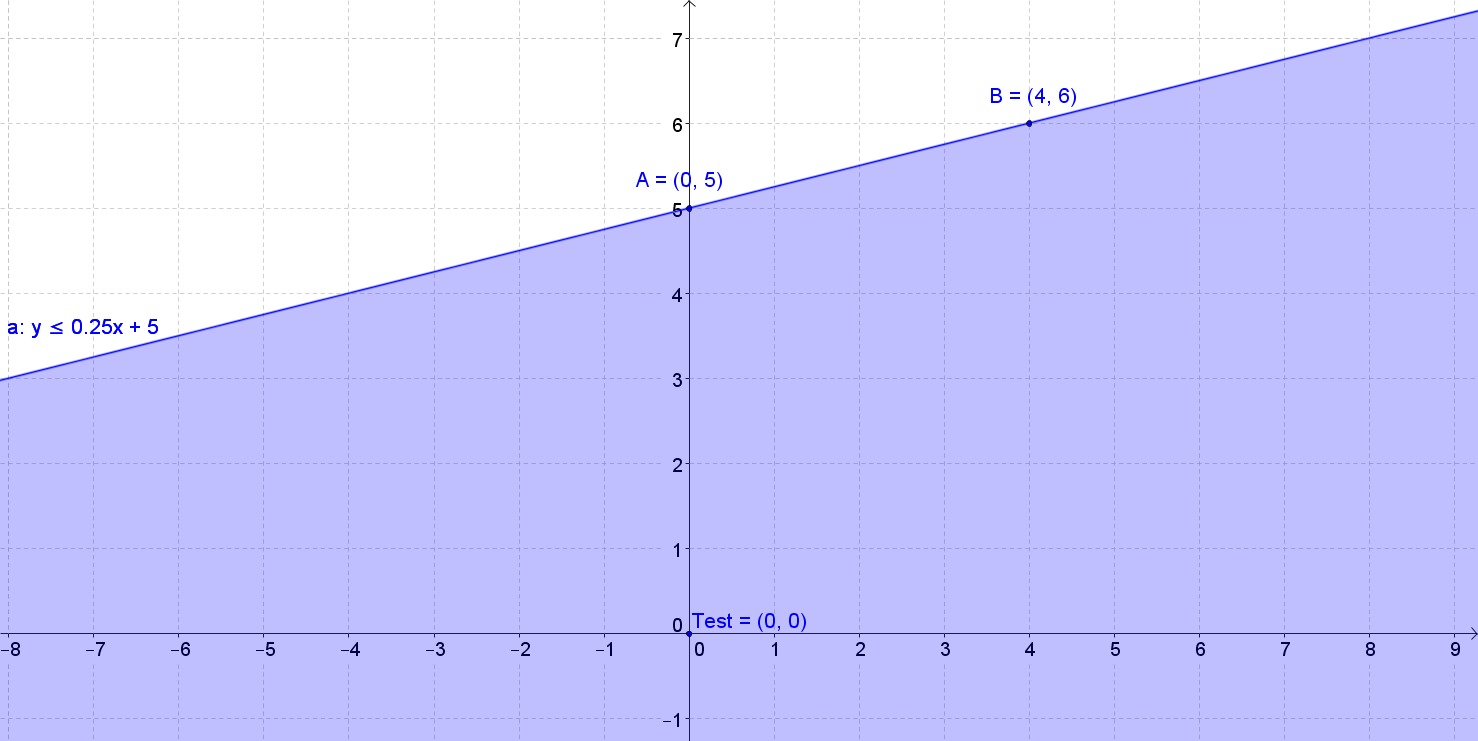Graph y ≥ 3x + 2
• Step 1:Graph the boundary line using the slope, m = 3 = [3/1] and the y - intercept (0,2). Starting at (0,2),
• go Up 3 and Right 1. If you don't prefer this method, you may create a table of values.
• The boundary line must be a solid line becasue you have the inequality ≤
• Step 2: Test the boundary using a point not on the line, the easiest point to test is (0,0). Always check that is not on the line, or else the test will be useless.
•  Test (0,0) 0 ≥ 3(0)+2 y ≥ 3x+2 0 ≥ 0+2 0 ≥ 2 Not True, shade away from (0,0) since (0,0) is not part of the solution set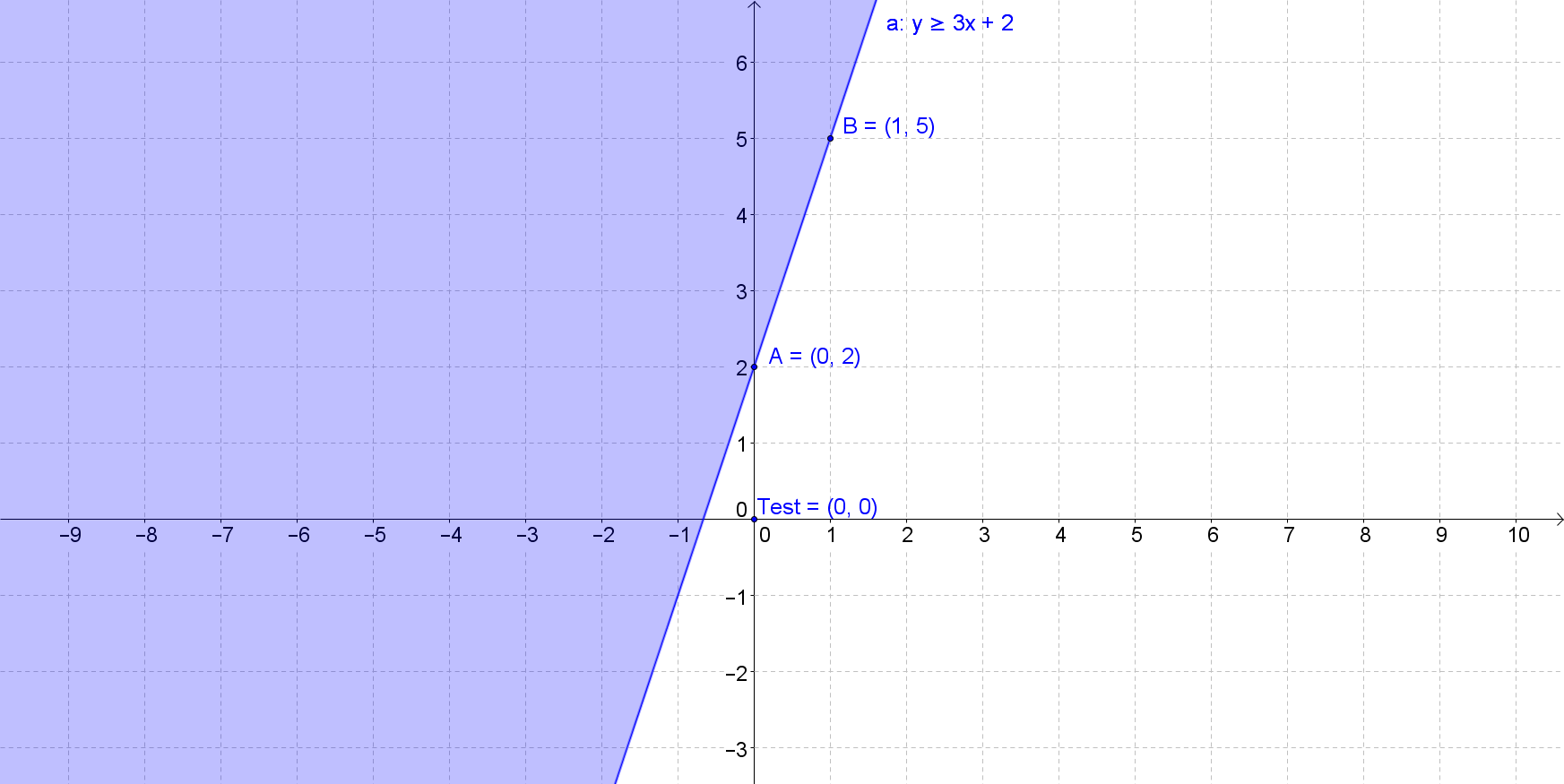Graph y <− [1/3]x − 2
• Step 1:Graph the boundary line using the slope, m = − [1/3] and the y - intercept (0, − 2).
• Starting at (0, − 2), go Down 1 and Right 3. If you don't prefer this method, you may create a table of values.
• The boundary line must be a dashed line becasue you have the inequality <
• Step 2: Test the boundary using a point not on the line, the easiest point to test is (0,0). Always check that is not on the line, or else the test will be useless.
•  Test (0,0) 0 < −[1/3](0)−2 y <−[1/3]x−2 0 < 0−2 0 < −2 Not True, shade away from (0,0) since (0,0) is not part of the solution set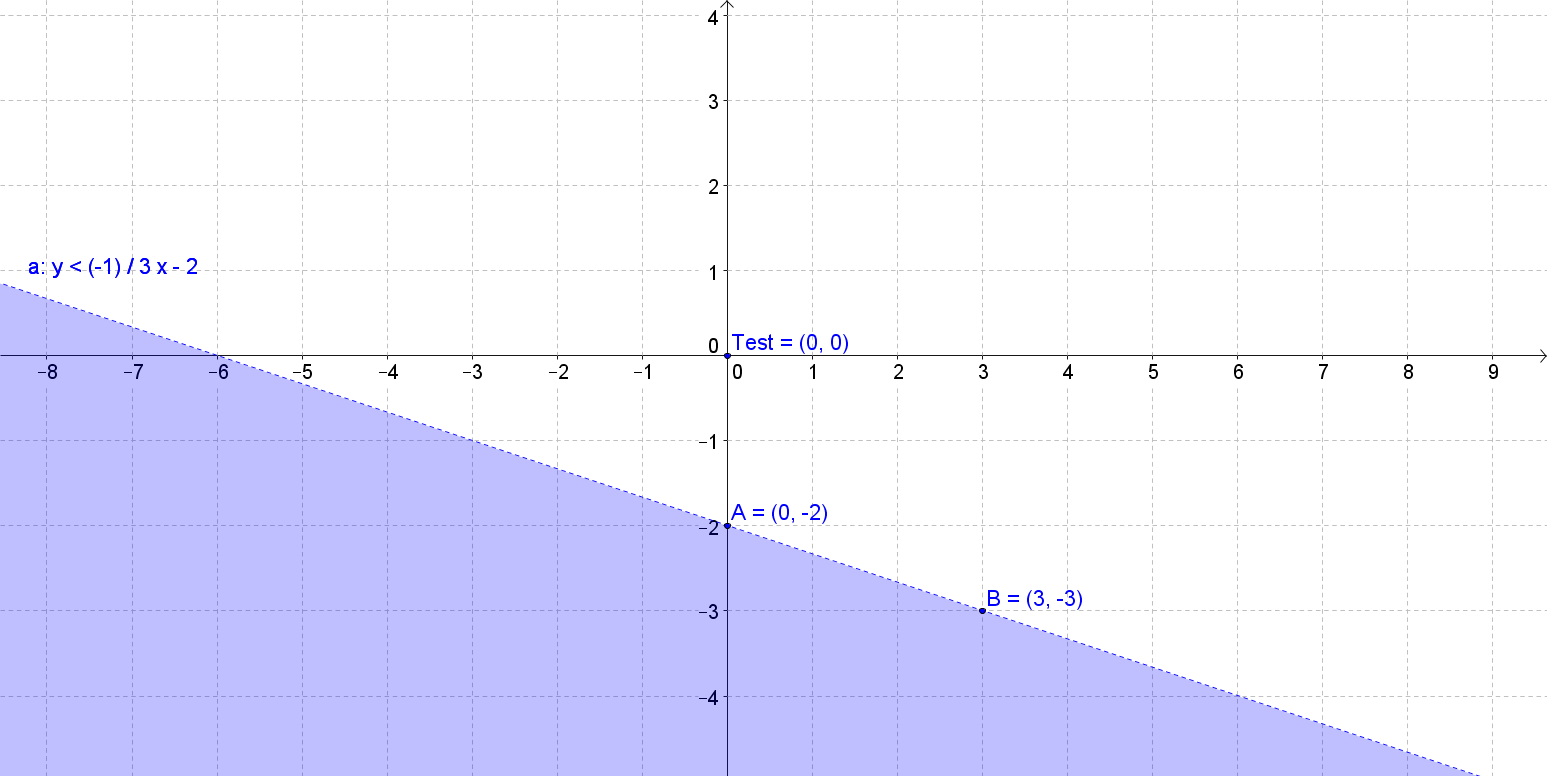Graph y > [3/2]x + 1
• Step 1 : Graph the boundary line using the slope, m = [3/2] = [3/2] and the y - intercept (0,1). Starting at (0,1), go Up 3 and Right 2. If you don't prefer this method, you may create a table of values.
• The boundary line must be a dashed line becasue you have the inequality >
• Step 2 : Test the boundary using a point not on the line, the easiest point to test is (0,0). Always check that is not on the line, or else the test will be useless.
•  Test (0,0) 0 > [3/2](0)+1 y < [3/2]x+1 y > 0+1 0 > 1 Not True, shade away from (0,0) since (0,0) is not part of the solution set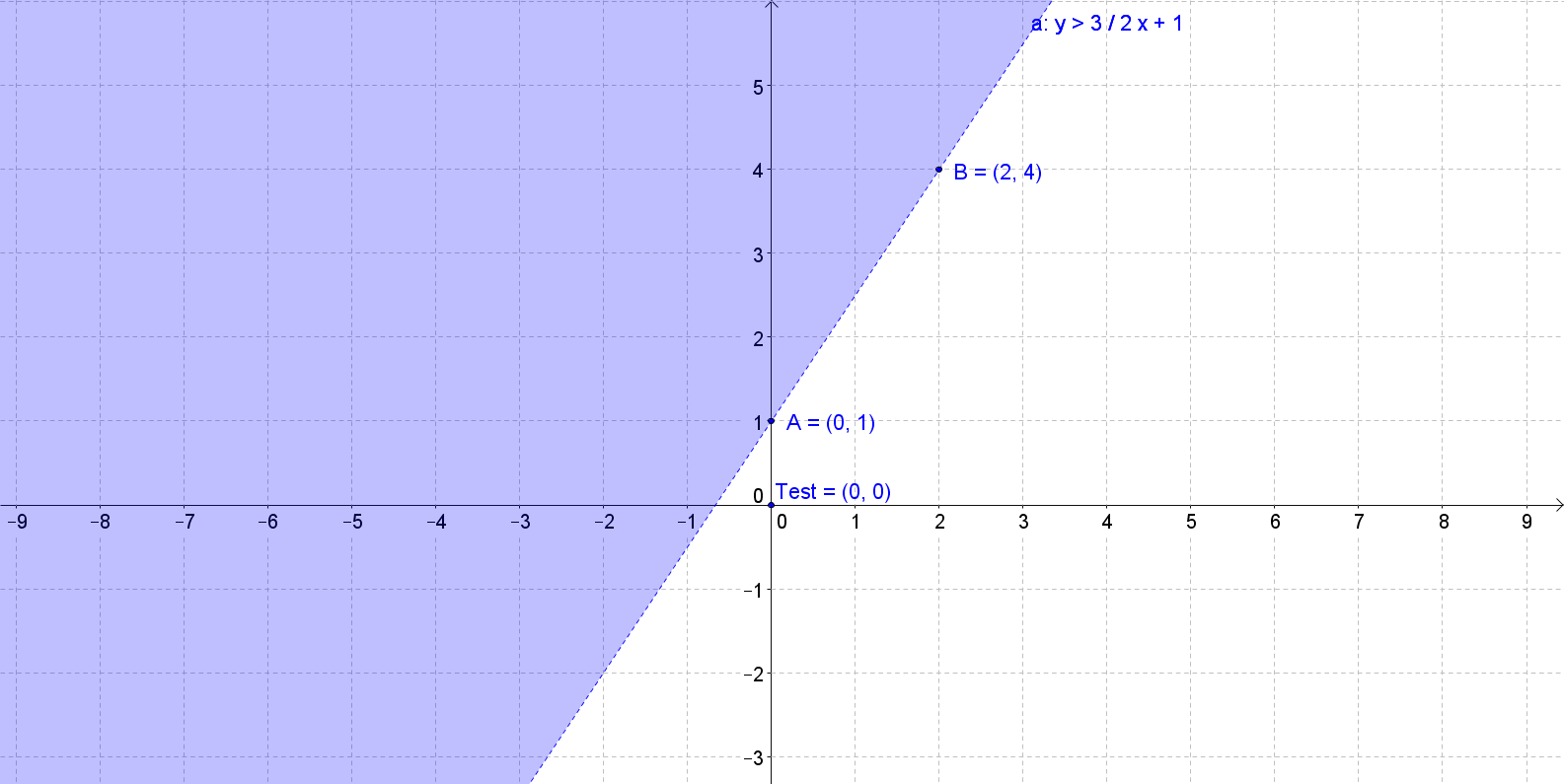Graph 5x + 2y > 10
• Step 1: Graph the boundary line by finding the x - and y - intercept. Boundary line will be a dashed line.
•  X-Intercept Y-Intercept Set y = 0 Set x = 0 5x + 2y = 10
•  X-Intercept Y-Intercept Set y = 0 Set x = 0 5x + 2y = 10 5x + 2(0) = 10 5(0)+2y = 0 5x = 10 2y = 10 x = 2 y = 5 (2,0) (0,5)
• Step 2: Test the boundary using a point not on the line, the easiest point to test is (0,0). Always check that is not on the line, or else the test will be useless.
•  Test (0,0) 5(0)+2(0) > 10 5x+2y > 10 0 > 10 Not True, shade away from (0,0) since (0,0) is not part of the solution set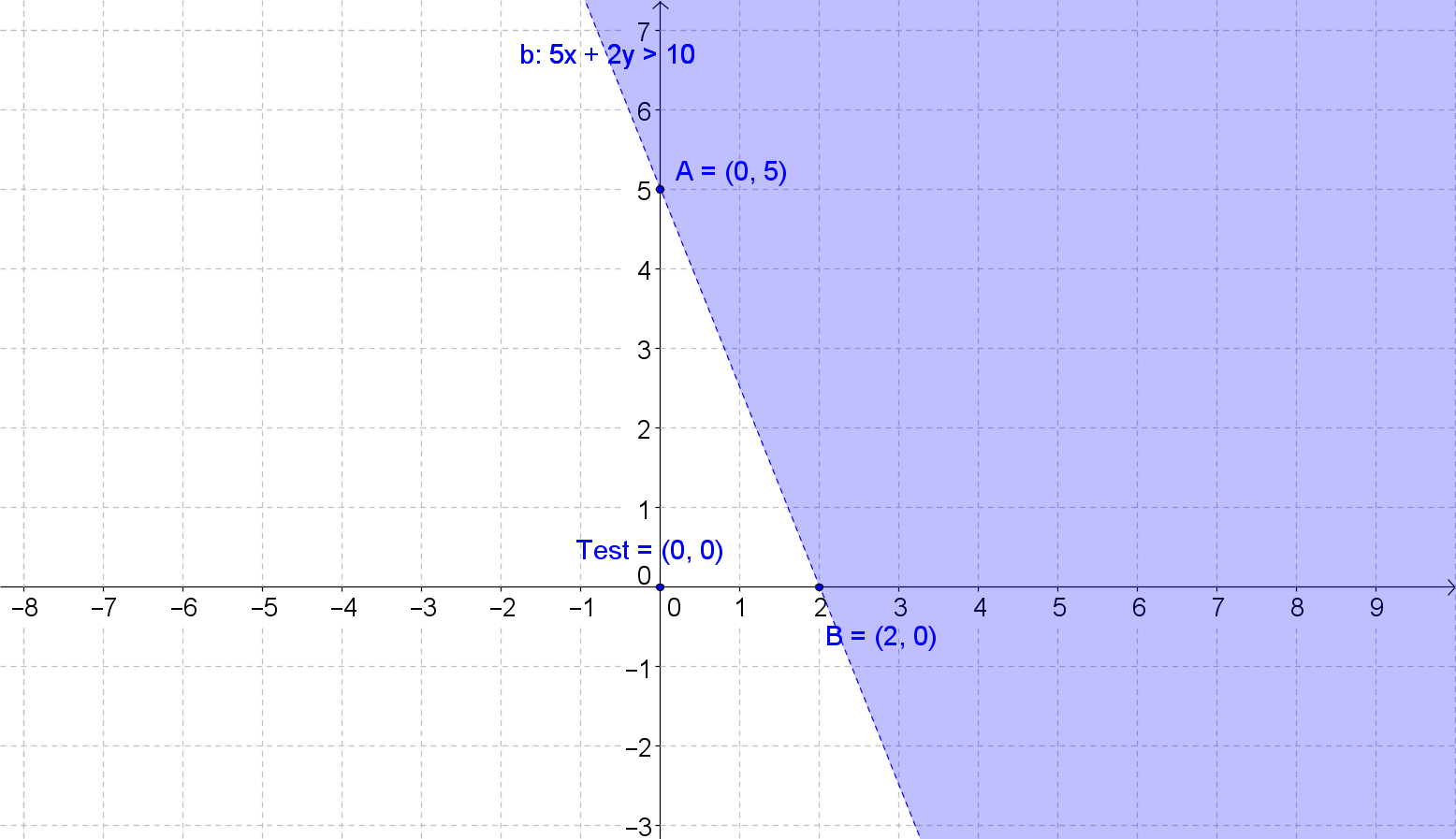Graph 2x + 3y < 6
• Step 1: Graph the boundary line by finding the x - and y - intercept. Boundary line will be a dashed line.
•  X-Intercept Y-Intercept Set y = 0 Set x = 0 2x+3y=6
•  X-Intercept Y-Intercept Set y = 0 Set x = 0 2x+3y=6 2x + 3(0) = 6 2(0) + 3y = 6 2x = 6 3y = 6 x = 3 y = 2 (3,0) (0,2)
• Step 2: Test the boundary using a point not on the line, the easiest point to test is (0,0). Always check that is not on the line, or else the test will be useless.
•  Test (0,0) 2(0) +3(0) < 6 2x+3y=6 0 < 6 True, shade towards (0,0) since (0,0) is part of the solution set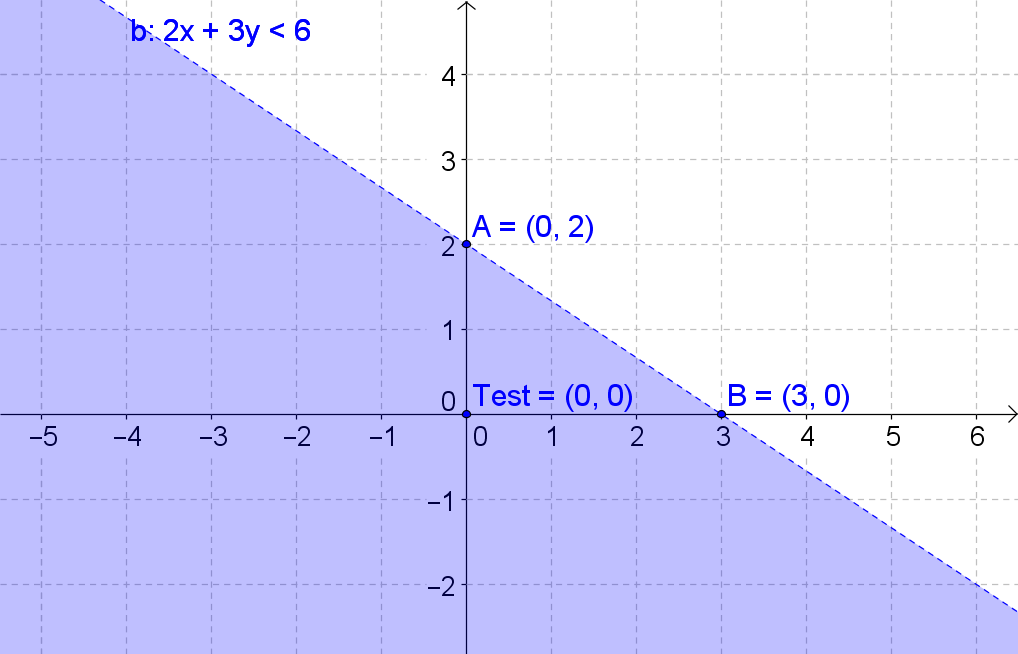Graph − 4x + y > 4
• Step 1: Graph the boundary line by finding the x - and y - intercept. Boundary line will be a dashed line.
•  X-Intercept Y-Intercept Set y = 0 Set x = 0 -4x+y=4
•  X-Intercept Y-Intercept Set y = 0 Set x = 0 -4x+y=4 -4x+0=4 -4(0)+y=4 -4x = 4 y=4 x = -1 (0,4) (-1,0)
• Step 2: Test the boundary using a point not on the line, the easiest point to test is (0,0). Always check that is not on the line, or else the test will be useless.
•  Test (0,0) −4x+y > 4 −4x+y=4 −4(0)+0 > 4 0 > 4 Not True, shade away (0,0) since (0,0) is not part of the solution set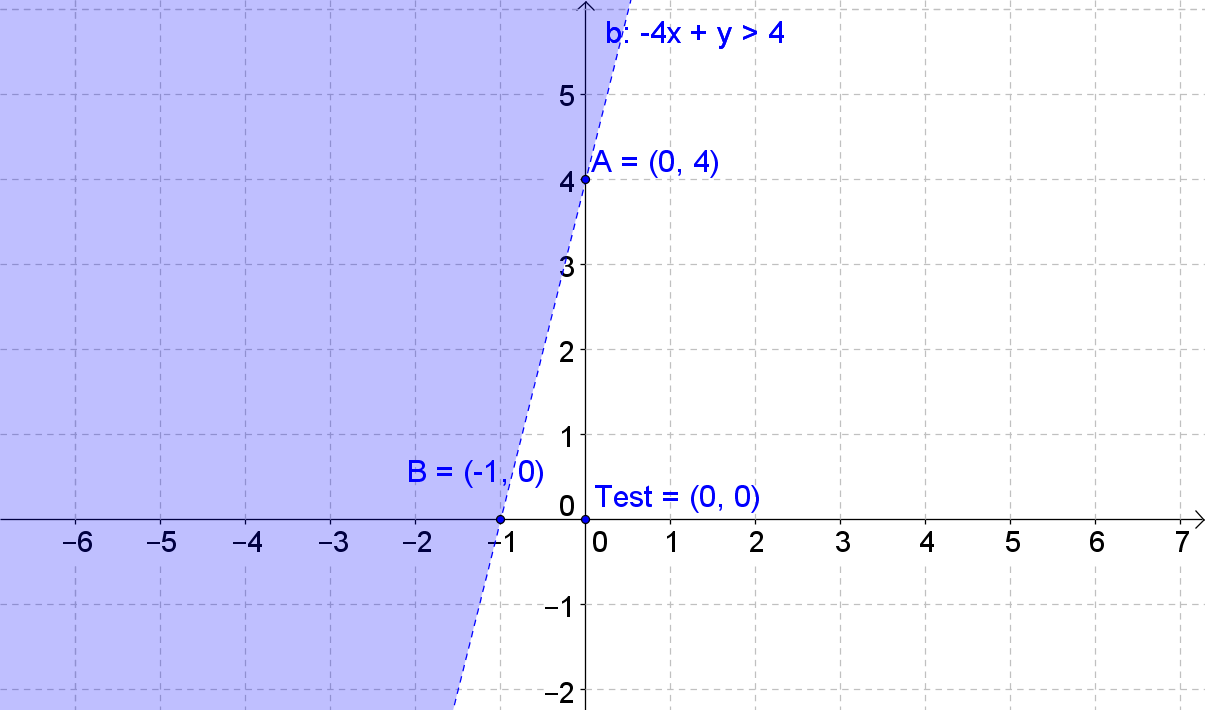Graph − 2x + 5y < 10
• Step 1: Graph the boundary line by finding the x - and y - intercept. Boundary line will be a dashed line.
•  X-Intercept Y-Intercept Set y = 0 Set x = 0 -2x+5y=10
•  X-Intercept Y-Intercept Set y = 0 Set x = 0 -2x+5y=10 -2x+5(0)=10 -2(0)+5y=10 -2x = 10 5y = 10 x = -5 y = 2 (-5,0) (0,2)
• Step 2: Test the boundary using a point not on the line, the easiest point to test is (0,0). Always check that is not on the line, or else the test will be useless.
•  Test (0,0) −2(0)+5(0) < 10 −2x+5y < 10 0+0 < 6 0 < 6 True, shade towards (0,0) since (0,0) is part of the solution set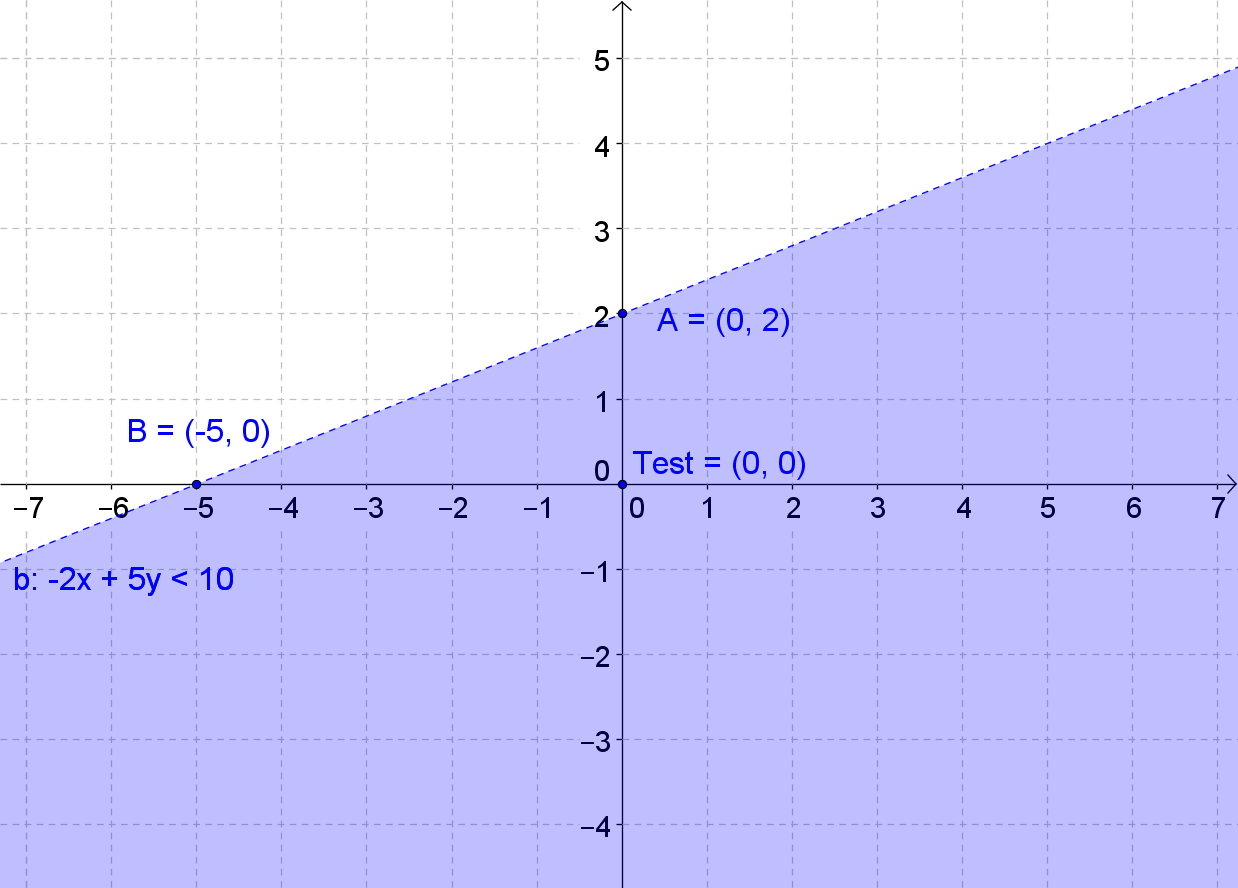Graph y >|x − 3| + 3
• Step 1: Graph the boundary line by completing a table of values.
•  x f(x) = - x-3 - + 3 0 1 2 3 4 5 6
•  x f(x) = |x−3| + 3 0 = |0 − 3| + 3 = 3 + 3 = 6 1 = |1 − 3| + 3 = 2 + 3 = 5 2 = |2 − 3| + 3 = 1 + 3 = 4 3 = |3 − 3| + 3 = 0 + 3 = 3 4 = |4 − 3| + 3 = 1 + 3 = 4 5 = |5 − 3| + 3 = 2 + 3 = 5 6 = |6 − 3| + 3 = 3 + 3 = 6
• Step 2: Test the boundary using a point not on the line, the easiest point to test is (0,0). Always check that is not on the line, or else the test will be useless.
•  Test (0,0) 0 > |0−3|+3 y > |x−3|+3 0 > 3+3 0 > 6 Not True, (0,0) is not part of the solution set Shade within the boundaries of the function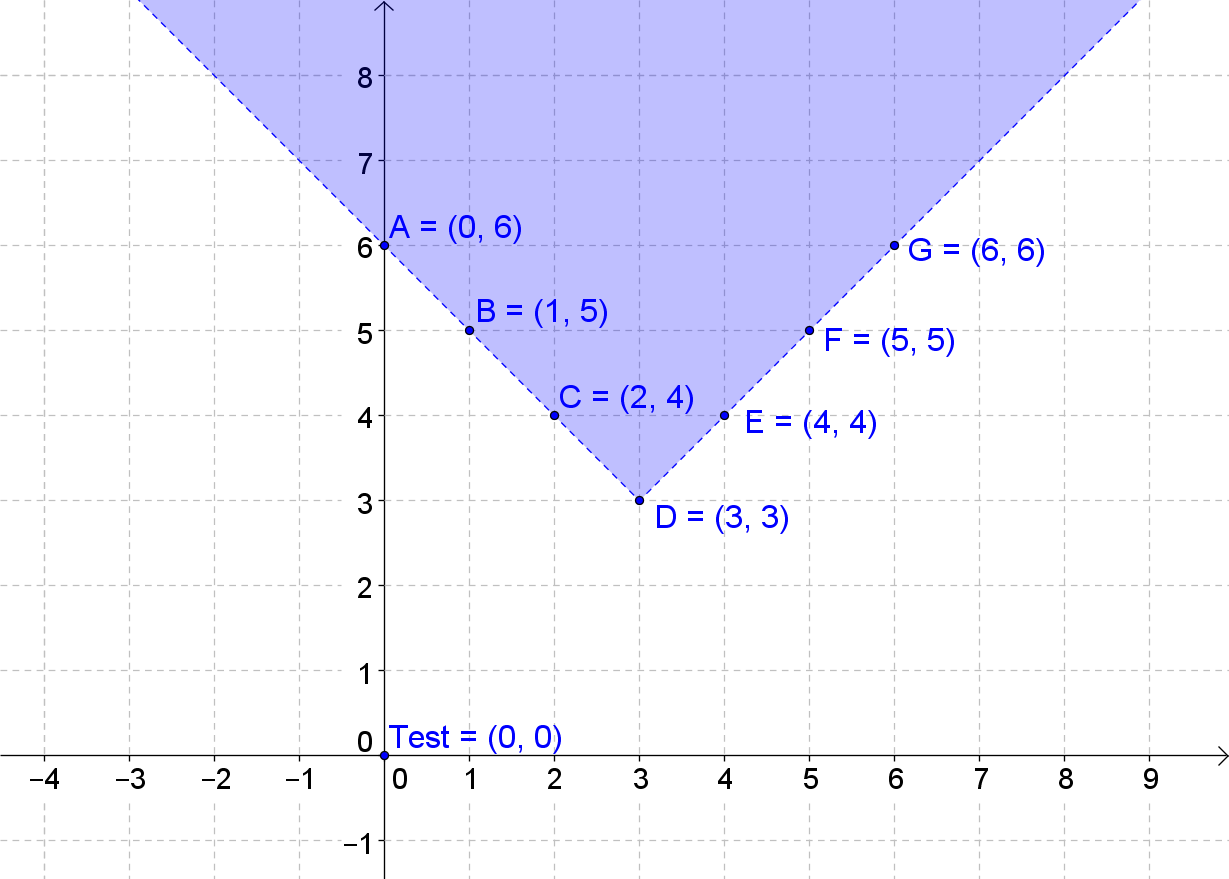Graph y <|x − 4| − 2
• Step 1: Graph the boundary line by completing a table of values.
•  x f(x) = y <|x − 4| − 2 1 2 3 4 5 6 7
•  x f(x) = y <|x − 4| − 2 1 = |1 − 4| − 2 = 3 − 2 = 1 2 = |2 − 4| − 2 = 2 − 2 = 0 3 = |3 − 4| − 2 = 1 − 2 = − 1 4 = |4 − 4| − 2 = 0 − 2 = − 2 5 = |5 − 4| − 2 = 1 − 2 = − 1 6 = |6 − 4| − 2 = 2 − 2 = 0 7 = |7 − 4| − 2 = 3 − 2 = 1
• Step 2: Test the boundary using a point not on the line, the easiest point to test is (0,0). Always check that is not on the line, or else the test will be useless.
•  Test (0,0) 0 <|0 − 4| − 2 y < |x−4|−2 0 < 4 − 2 0 < 2 True, (0,0) is part of the solution set Shade outside the boundaries of the function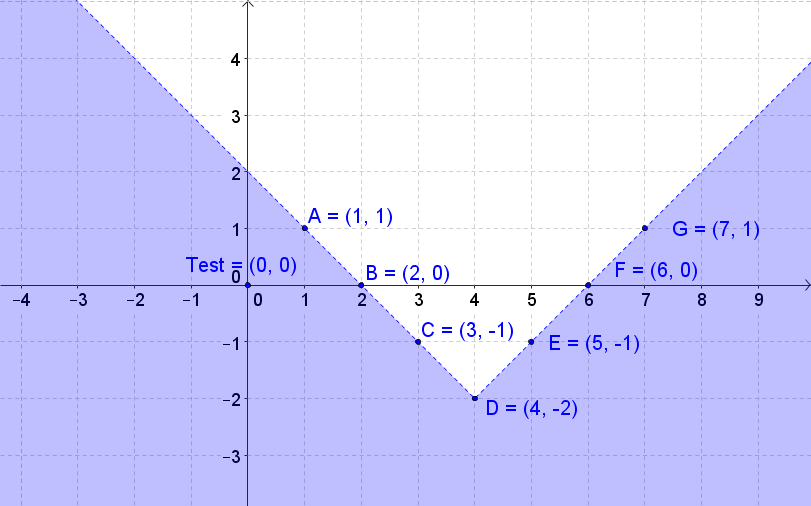*These practice questions are only helpful when you work on them offline on a piece of paper and then use the solution steps function to check your answer.

### Graphing Inequalities

Lecture Slides are screen-captured images of important points in the lecture. Students can download and print out these lecture slide images to do practice problems as well as take notes while watching the lecture.

• Intro 0:00
• Graphing Linear Inequalities 0:07
• Using Test Points
• Graph Corresponding Linear Function
• Dashed or Solid Lines
• Use Test Point
• Example: Linear Inequality
• Graphing Absolute Value Inequalities 4:50
• Graph Corresponding Equations
• Use Test Point
• Example: Absolute Value Inequality
• Example 1: Linear Inequality 9:17
• Example 2: Linear Inequality 11:56
• Example 3: Linear Inequality 14:29
• Example 4: Absolute Value Inequality 17:06

### Transcription: Graphing Inequalities

Welcome to Educator.com.0000

In today's lesson, we are going to be graphing inequalities.0002

Recall that, in order to graph a linear inequality, you have to take a series of steps.0008

And the graph of a linear inequality is a shaded region in the coordinate plane.0014

And those shaded regions are known as half-planes; the boundary of the region, or the half-plane, is a straight line,0019

which is the graph of the corresponding linear function.0027

The region, or the half-plane, containing the solution set can be determined by using a test point.0032

OK, so the steps in order to graph a linear inequality are to first graph the corresponding linear function; and that is a straight line.0040

And that line is dashed if the inequality you are working with is a strict inequality (less than or greater than).0058

The line is solid if you are dealing with less than or equal to, or greater than or equal to; and we will talk in a minute about what that means.0071

After you have graphed the line, then you end up with the coordinate plane divided into two half-planes.0079

You use a test point to determine the region containing the solution set.0097

So, I am going to go ahead and do an example now: but if you need more review on this, check out the Algebra I video on this topic.0104

All right, the easiest way to understand is to look at an example: let's look at the inequality y - 3x < -2.0112

My first step is to graph the corresponding linear function, which would be y - 3x = -2.0123

So, I am going to go ahead and find some x-values and some y-values--some coordinate pairs on this line.0132

When x is 0, y is -2; so that gives me the y-intercept.0140

When x is 1, this is going to give me -3; add 3 to both sides--y will be 1.0145

When x is 2, -3 times 2 is -6; add that to both sides, and y is 4.0155

All right, I have enough to graph the line: x is 0, y is -2--the y-intercept; then I have another point at (1,1) and another point at (2,4).0164

Because this is a strict inequality, I am going to use a dashed line.0177

This line divides the coordinate plane into two half-planes: an upper and lower half-plane.0187

One of these half-planes contains the solution set for the inequality; the way I figure out which one is to use a test point.0193

Pick a point that is not on the boundary line, and that is easy to work with.0201

(0,0) is very easy to work with; so I am going to take my test point, and I am going to go back to my inequality and plug it in.0206

I am going to see what happens: this gives me 0 - 0 < -2; 0 is less than -2--that is not true.0216

What this means is that this is not part of the solution set.0230

If this is not part of the solution set, it is in the half-plan that is not contained in the solution set.0234

Therefore, the solution set is actually in the lower half-plane.0240

And you could verify that by checking a point down here.0245

The idea is to graph the corresponding linear function to find the boundary line.0249

Now, I mentioned that this is a strict inequality, so I am using a dashed line.0253

And what that means is that the boundary line is not part of the solution set.0256

If it were a solid line (because I had less than or equal to), then the boundary line would be part of the solution set.0262

But actually, in this, the solution set is just below that boundary line.0268

I determined which half-plane was correct by using a test point.0272

Since the test point ended up giving me an inequality that is not true, that is not valid,0276

I knew that that test point did not lie in the half-plane of the solution set, so I shaded in the lower half-plane.0281

Graphing absolute value inequalities: the procedure for these inequalities is similar to the procedure for linear inequalities.0290

So, we are going to use the same technique.0298

Recall that the technique involved first graphing the line for the corresponding equation.0302

Graph the corresponding equation: in this case, it is going to be an absolute value equation.0309

And then, use a test point to determine the half-plane containing the solution set for the inequality.0318

All right, let's take an example: y ≤ |x-3|.0338

The corresponding equation would be y = |x-3|, so I need to graph that to find my boundary.0347

So, some values for x and y: when x is -2, -2 minus 3 is -5; the absolute value is 5.0357

When x is -1, this becomes -4; the absolute value is 4; when x is 0, this becomes -3; the absolute value is 3.0368

Let's jump up to 3: 3 minus 3 is 0--the absolute value is 0.0381

4 minus 3 is 1; the absolute value is 1; 5 minus 3 is 2--the absolute value is 2.0389

OK, plotting these points: first, (-2,5), (-1,4), (0,3), (3,0), (4,1), (5,2); OK, I have my typical v-shaped graph of absolute value.0399

And you notice that I made this a solid line; and the reason that is a solid line is because I had less than or equal to.0439

So, this boundary is actually going to be part of the solution set.0447

OK, so I graphed the corresponding equation, and I determined that it is a solid line.0451

Now, I need to determine if the solution set is down here, or if it is above the boundary; is it above or below the boundary line?0455

So, I am going to use a test point of (0,0): that is going to be my test point.0462

I go back to the inequality, and I see what happens when I put my test point values in there.0468

This gives me 0 ≤ |-3|; that would say that 0 is less than or equal to 3; that is true.0477

Since that is true, what that tells me is that this is part of the solution set.0489

(0,0) is part of the solution set, and that means that this lower half-plane where (0,0) is, my test point, contains the solution set.0495

So, as I mentioned, this is very similar to the technique for graphing linear inequalities.0505

First, graph the corresponding equation; and use a solid line if it is less than or greater than or equal to.0510

Use a dashed line if it is a strict inequality (just greater than or less than).0520

So then, once you have your boundary line, find a test point that is off the boundary line, and not so close to it as to cause any confusion.0525

And choose one that is simple: (0,0), (1,1)--very easy to work with.0533

Plug that into the absolute value inequality; and if you come out with a true inequality,0538

like this one, you know that you are in the correct area with the solution set.0544

If you come out with a statement or inequality that is not valid, you shade in the other region.0548

OK, the first example: we have an inequality that we are asked to graph: y ≤ 2x - 3.0557

My first step is going to be to graph the corresponding linear equation, in order to determine the boundary.0567

Some values for x and y: when x is 0, y is -3; when x is 1, 1 times 2 is 2, minus 3 is -1.0581

When x is 2, 2 times 2 is 4, minus 3 is 1.0594

OK, so plotting this out: (0,-3), (1,-1), (2,1); I have plotted this boundary line, and I look up here,0599

and I see that it is less than or equal to; so I am going to make this a solid line.0615

It is going to be a solid line: this line will be part of the solution set.0621

So, first I graph the boundary; next, I am going to look at a test point.0626

OK, I can again use this very simple test point of (0,0), the origin.0638

Going back to the inequality to look at my test point, y ≤ 2x -3; the test point is (0,0), so y is 0; x is 0.0646

This is saying that 0 is less than or equal to -3; that is not true--this is not in the solution set.0664

Well, the origin is up here; this means that this whole area is not part of the solution set.0678

So, I am going to go to the lower half-plane and shade that in to indicate that this shaded region, including the boundary line, is the solution set.0684

Again, the first step is to take the corresponding linear equation and graph it.0692

The second step: find a test point that is easy to work with and away from the boundary line, and plug that in.0698

If you come up with an inequality that is not true, like this, shade the other half.0705

If it is true, shade the half containing the test point.0711

Example 2: We are graphing another inequality, 2x + y > 6.0716

First, I am going to find the corresponding equation: 2x + y = 6.0724

I am going to keep this simple: I am going to use the intercept method in order to graph this.0733

I am going to let x equal 0 to find the y-intercept: when x is 0, y is 6.0738

Then, I am going to let y equal 0 to find the x-intercept: when y is 0, then I am going to end up with 2x + 0 = 6, or 2x = 6; x = 3.0745

So, I have my x and y intercepts; it is going to give me...when x is 0, y is 6, right about there; when x is 3, y is 0.0759

OK, then I look, and I have a strict inequality, so I am going to have a dashed line;0771

this line is going to be dashed, and is not going to be part of the solution set.0777

So, I found my boundary line; and that is my first step; my second step is going to be to work with my test point.0788

The test point is right here: it is the origin, (0,0).0798

Going back and looking at the inequality: 2 times 0, plus 0, is greater than 6; 0 plus 0 is greater than 6.0803

Well, 0 is not greater than 6, so this is not true--not in the solution set.0817

The origin is not part of the solution set, so the lower half-plane is not the correct half-plane; I am going to shade, instead, this upper half-plane.0830

Again, graph the linear equation corresponding to the inequality.0839

Since this is strict inequality, plot that line using a dashed line, since this boundary is not part of the solution set.0844

Find a test point; the origin is a really good one, as long as it is not on the boundary line or very close to the boundary line.0853

Substitute (0,0) into the inequality; and you come up with an inequality that is not true.0859

Example 3: Graph 3x - 4 > y.0870

First, graph the boundary line: the corresponding equation is 3x - 4 = y.0876

To graph that, I am going to need some x and y values; when x is 0, y is -4; when x is 1, that gives me 3 - 4 = y, so y equals -1.0889

One more value: when x is 2, y is 2; OK.0908

So, I have enough information to plot my line: when x is 0, y is -4; (1,-1), (2,2).0918

The next issue: strict inequality--that means this is a dashed line.0935

OK, so now, I have this boundary; I have an upper and a lower half-plane; and I need to figure out where my solution set is.0948

The origin is well away from the boundary line, so I can use that as my test point.0956

So, test point is (0,0): the inequality is 3x - 4 > y; and this gives me 0 - 4 > 0, which comes out to -4 > 0.0963

And that is, of course, not true; this is not in the solution set, because this inequality did not hold true.0986

Therefore, I am going to go to the other half-plane, and I am going to shade that in.0997

I graphed the boundary line using a dashed line, since it is a strict inequality.1007

And then, I took a test point, substituted those values into the inequality, and determined that the origin (0,0) is not part of the solution set.1011

So, my other half-plane contains the solution set.1022

Example 4: we are working with an absolute value inequality where y is less than the absolute value of x - 2, plus 1.1027

I am starting out with just thinking that I expect this to look like a v, because absolute value graphs are v-shaped.1036

I am graphing the corresponding equation, y = |x - 2| + 1.1046

And with absolute value inequalities or equations, I like to get more values,1051

because I want to make sure that I don't end up missing half of my v.1055

So, when x is -2, that is going to give me -4; the absolute value of that is 4; plus one is 5.1061

-1 minus 2 is -3; the absolute value is 3, plus one is 4.1070

0 minus 2 is -2; the absolute value is 2, plus one is 3.1077

Now, some positive numbers: 1 minus 2 is -1; the absolute value is 1; plus one is 2.1085

2 minus 2 is 0, plus one is 1; 3 minus 2 is 1; the absolute value is 1, plus one is 2.1094

4 minus 2 is 2; the absolute value is 2, plus one is 3; and now I have enough values to work with.1111

So, (-2,5), (-1,4), (0,3), (1,2), (2,1), (3,2)...so you see the v forming, so I know I have enough points,1119

because I have both parts of my v...(4,3).1145

It's a strict inequality, so I am using a dashed line.1150

And you see that it is the typical v shape, but it is shifted over.1161

And I have a -2 here, and so this is actually shifted over to the right by 2; and plus 1, so it is shifted up by 1.1165

I found the boundary; again, I am fortunate--I can easily use (0,0) as the test point--the origin.1173

Going back to the inequality: this is going to be 0 < |0 - 2| + 1.1187

So, that is 0 < |-2| (the absolute value of -2 is 2) + 1, so this gives me 0 < 3.1201

0 actually is less than 3; therefore, the origin is part of the solution set, so this is a true statement, and the origin is part of the solution set.1219

(0,0) is part of the solution set, and that means that I am in the correct half-plane, and I am going to go ahead and shade that in.1240

OK, so we handled this just with a very similar technique to linear inequalities.1257

Graphing out the absolute value equation, I got my v-shaped graph with a dashed line for the strict inequality.1263

I used a test point, (0,0), substituted that into the inequality, and found that I had a true statement--a true inequality, 0 < 3.1270

Since that is true, I knew that the origin lay within the region that contains the solution set.1279

So, I went ahead and shaded that region to note that my solution set is located there.1289

That concludes this lesson of Educator.com; see you next lesson!1297

OR

### Start Learning Now

Our free lessons will get you started (Adobe Flash® required).